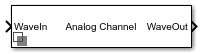Documentation

# Analog Channel

Construct loss model from channel loss metric or impulse response

• Library:
• SerDes Toolbox / Utilities## Description

The Analog Channel block constructs a loss model using a channel loss metric or an impulse response from another source in a SerDes Toolbox™ model. Analog model inputs are only used for IBIS file construction when using impulse response. For more information, see Analog Channel Loss in SerDes System.

## Ports

### Input

expand all

Input signal, specified as a waveform.

Data Types: `double`

### Output

expand all

Modified output data that includes the effect of a lossy printed circuit board transmission line model according to the method outlined in .

Data Types: `double`

## Parameters

expand all

#### Channel Model

Source of channel model.

• Select `Loss model` to model the analog channel from a loss model.

• Select `Impulse response` to model the analog channel from an impulse response.

#### Programmatic Use

• Use `get_param(gcb,'ChannelType')` to view the current Channel model.

• Use `set_param(gcb,'ChannelType',value)` to set a specific Channel model.

Frequency for the desired channel loss, specified as a positive real scalar in Hz. It corresponds to the Nyquist frequency of the system.

#### Dependencies

This parameter is only available when `Loss model` is selected as Channel model.

#### Programmatic Use

• Use `get_param(gcb,'TargetFrequency')` to view the current value of Target frequency (Hz).

• Use `set_param(gcb,'TargetFrequency',value)` to set Target frequency (Hz) to a specific value.

Data Types: `double`

Channel loss at the target frequency, specified as a scalar in dB.

#### Dependencies

This parameter is only available when `Loss model` is selected as Channel model.

#### Programmatic Use

• Use `get_param(gcb,'Loss')` to view the current value of Loss (dB).

• Use `set_param(gcb,'Loss',value)` to set Loss (dB) to a specific value.

Data Types: `double`

Characteristic impedance of the channel, specified as a positive real scalar in ohms. Impedance (Ohms) depends on the setting of Signaling in the Configuration tab in the SerDes Designer app or in the Configuration block.

• If Signaling is set to `Differential`, the default value of Impedance (Ohms) is `100`.

• If Signaling is set to `Single-ended`, the default value of Impedance (Ohms) is `50`.

#### Dependencies

This parameter is only available when `Loss model` is selected as Channel model.

#### Programmatic Use

• Use `get_param(gcb,'Zc')` to view the current value of Impedance.

• Use `set_param(gcb,'Zc',value)` to set Impedance to a specific value.

Data Types: `double`

User provided impulse response, specified as a unitless vector. Impulse response is used to construct a channel loss model from the user-defined impulse response of the system.

#### Dependencies

This parameter is only available when `Impulse response` is selected as Channel model

#### Programmatic Use

• Use `get_param(gcb,'ImpulseResponse')` to view the current value of Impulse response.

• Use `set_param(gcb,'ImpulseResponse',value)` to set Impulse response to a specific value.

Data Types: `double`

#### Analog Model

Single-ended impedance of the transmitter analog model, specified as a nonnegative real scalar in ohms.

#### Programmatic Use

• Use `get_param(gcb,'TxR')` to view the current value of Tx R (Ohms).

• Use `set_param(gcb,'TxR',value)` to set Tx R (Ohms) to a specific value.

Data Types: `double`

Capacitance of the transmitter analog model, specified as a nonnegative real scalar in farads.

#### Programmatic Use

• Use `get_param(gcb,'TxC')` to view the current value of Tx C (F).

• Use `set_param(gcb,'TxC',value)` to set Tx C (F) to a specific value.

Data Types: `double`

Single-ended impedance of the receiver analog model, specified as a nonnegative real scalar in ohms.

#### Programmatic Use

• Use `get_param(gcb,'RxR')` to view the current value of Rx R (Ohms).

• Use `set_param(gcb,'RxR',value)` to set Rx R (Ohms) to a specific value.

Data Types: `double`

Capacitance of the receiver analog model, specified as a nonnegative real scalar in farads.

#### Programmatic Use

• Use `get_param(gcb,'RxC')` to view the current value of Rx C (F).

• Use `set_param(gcb,'RxC',value)` to set Rx C (F) to a specific value.

Data Types: `double`

20%−80% rise time of the stimulus input to transmitter analog model, specified as a positive real scalar in seconds.

#### Programmatic Use

• Use `get_param(gcb,'RiseTime')` to view the current value of Rise time (s).

• Use `set_param(gcb,'RiseTime',value)` to set Rise time (s) to a specific value.

Data Types: `double`

Peak-to-peak voltage at the input of transmitter analog model, specified as a positive real scalar in volts.

#### Programmatic Use

• Use `get_param(gcb,'VoltageSwingIdeal')` to view the current value of Voltage (V).

• Use `set_param(gcb,'VoltageSwingIdeal',value)` to set Voltage (V) to a specific value.

Data Types: `double`

 IEEE 802.3bj-2014. "IEEE Standard for Ethernet Amendment 2: Physical Layer Specifications and Management Parameters for 100 Gb/s Operation Over Backplanes and Copper Cables." URL: https://standards.ieee.org/standard/802_3bj-2014.html.

##### SupportGet trial now